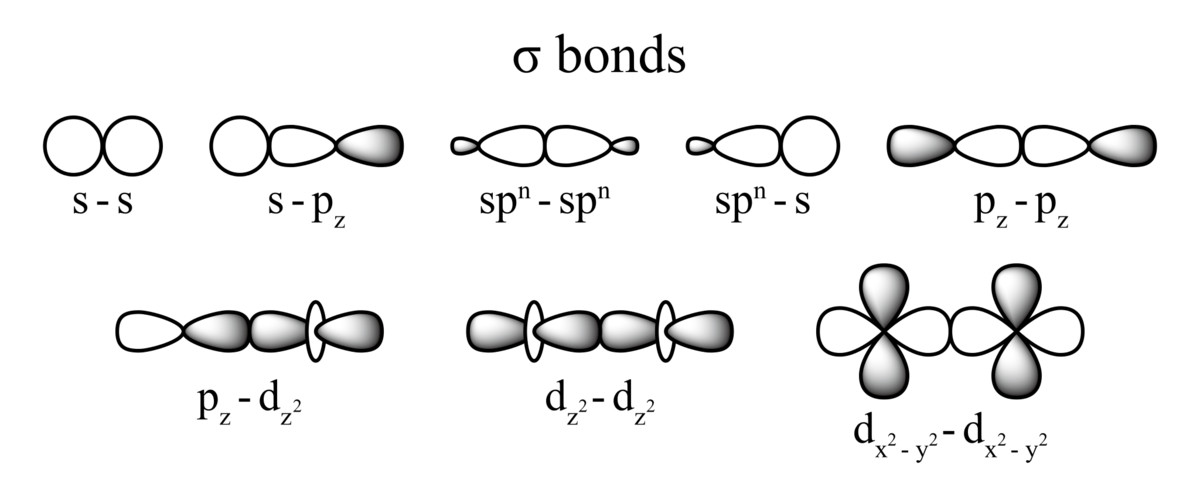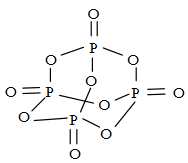#### Number of sigma bonds inis Option 1)Option 2)Option 3)Option 4)As we learnt in

Sigma bond -

When covalent bond is formed by overlapping of atomic orbitals along the same axis it is called Sigma bond. Such type of bond is symmetrical about the line joining the two nuclei.

- whereinNo ofbondsNo ofbondsThe number of sigma bond in P4O10 is 16

Option 1)The solution is incorrect

Option 2)The solution is incorrect

Option 3)The solution is incorrect

Option 4)The solution is correct

#### Plabita